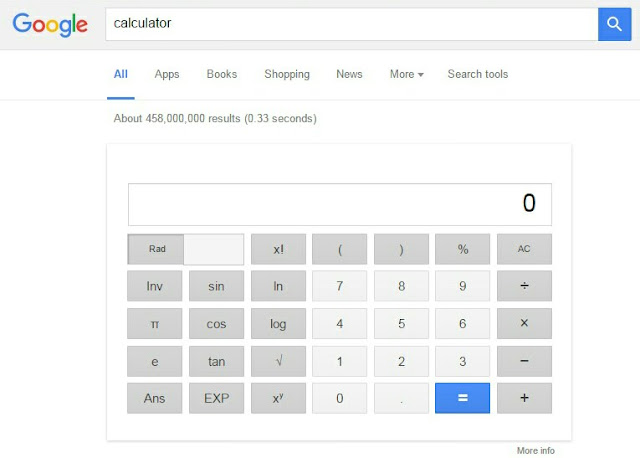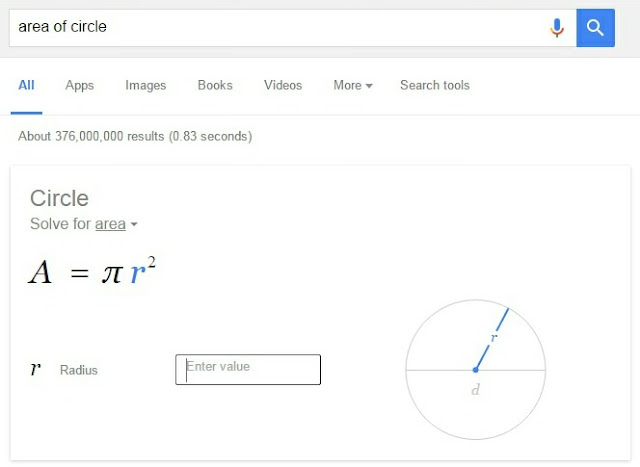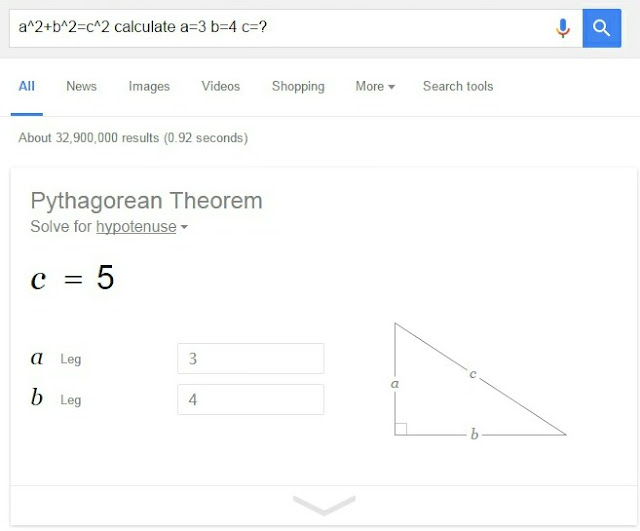# How to Use Google as a Calculator and Unit Converter

Good thing about Google is that, it's fast, accurate and easy to use. This is why it's most used and even most loved website. I might not be wrong If I will say that Google is much more than search engine. It has thousands of features/products and one of them is Google's calculator.Yes, Google has its own calculator which you solve all your basic maths calculations. Now you don't need to keep any calculator in your pocket. Just open Google and ask your problems. It will give you accurate answers. Apart from basic calculations, you can also convert one unit into another. There are few essential tips and tricks related to this calculator. In this tutorial, I will guide you how to use it in right way.

## Meet the Google Calculator & Unit Converter

Google calculator can solve all basic arithmetic, geometric, logarithmic and trigonometric calculations. On the other hand, it can also find out the value of constants. You can also use it to convert units. Here's a complete guide -

### 1. Nuclear option: search "calculator"

In this method, you don't have do anything. Simply open Google homepage and then search for "calculator" (without quotes). This will show you the basic calculator before normal search results (SEPR) as shown in below picture.Now solve your calculations as like solve them in other calculators. You can also use trigonometric and log functions. Unfortunately, this calculator will not work in mobile browsers (even If you've Android phone). It is available only for desktop version of Google.

However, there is simply tricks to access it. If you're using Android phone, open Google Chrome and then click on option menu button (three dots). From menu list, find and tick mark "Request desktop site" option. After this, try to open Google and you'll be entered in desktop version automatically. Now search "calculator" again and it will definitely work this time.

### 2. Solve basic arithmetic problems

Although you can solve basic arithmetic calculations from first method but there is another manual way to do this. This will work in almost all browsers and different site versions. You just need to type the values and add operator between them. There are four basic arithmetic operators which are plus (+) for addition, negative (-) for subtraction, asterisk (*) for multiplication and slash (/) for division.

If you'll search for "2+2=", you'll get back the answer 4. This statement can be written as "What is 2+2" or "what is the sum of two plus two". It doesn't matter whether you type it in words or numbers, both will give you the same results. Here are few more example -

• (12+10)/20 - This will give you 1.1 as an answer.
• what is 10000000/0 - No answer found as anything divided by 0 is not defined.
• (12*30)/(12*40) - The answer will be 0.75.
• ((1+1)*3)/((1*4)-1) - This answer will be 2 but make sure to put necessary terms under brackets otherwise it may cause conflict.
Now lets talk about exponents and roots. To find the exponents, add ^ symbol to the power. For example, "2^4" means 4 times 2 which is equal to "2*2*2*2" and you'll get back the 16 answer. You can also add decimal values in exponent like "10^2.5".

For roots, it's quite easy. Simple type "square roots of 2" and it will give you the exact value which is 1.41421356237. In the same way, you can find cube, fourth, fifth and other roots as well. For example, "10th root of 100" will give 1.58489319246 answer. Try more complicated calculations yourself.

### 3. Find constant numbers

The most irritating thing about physics, chemistry and mathematics is that, it is really hard to remember the values of constant. There are hundreds of constants. Even If you'll remember them for your exams, you'll definitely forget them in future within 1 or 2 weeks. Well there is good news for all. Google calculator can help you find out all constant values easily. For example, If you'll search "what is the value of pi", it will return 3.14159265359 answer. Here are few more common example -
• what is the value of astronomical unit - Astronomical is the unit of distance and it's equally to 149597871 kilometers, which is the distance between the sun and the earth.
• what is the value of Planck constant - The answer will be 6.626176 x 10-34 joule-seconds.
• the speed of light - Google will answer the accurate value which is 299 792 458 m/s. You can also find the speed of light in different mediums.### 4. Solve geometry calculations

Google calculator can solve all basic geometry calculations. If you'll type "area of circle", it will show you the preview as shown in below picture.Now just enter the value of radius and it will automatically calculate the answer within second.

Note: The preview shown in above picture will not work in mobile browsers. So again you can request desktop site in Google Chrome if you're using your Android phone.

Now lets see another example for triangles. Search "area of triangle" and Google will show the image of the triangle with formula. Enter the values of base and perpendicular and it will calculate the right answer.

Another interesting thing is that, you can also find unknown values. For example, search this - "a^2 + b^2 = c^2 calculate a=4, b=3, c=?". As you can see, it is the Pythagorean Theorem where base and height are 4 and 3 and c represents hypotenuse. The answer will be 5 (See below picture). You can also try this trick for cone, cylinder, sphere and other geometry shapes.### 5. Find log & trigonometric values

Log and trigonometry is used everywhere from maths calculations to physics and chemistry numericals. Though it's a very big headache to remember and calculate their values but now Google can help you the find out them at your fingertips. Here are some examples -
• log(1) - The answer will be 0.
• sin of 30 degree - It's 0.5.
• sin(pi/2)+cos(pi/2) - You can also add arithmetic operators between them. Here answer is 1.
• log(10)*pi - As log(10) is 1, so the whole term is equal to the value of pi.
Note: If you'll search "sin(30)", the answer will not be 0.5, because Google assume that the value under brackets is in radian. That's why I had mentioned degree and pi words in above examples.

### 6. Convert units

Last but not least, Google can easily convert one unit into another. It is very simple. Type something like this - "1100m in km" and it will convert meters in kilometers (which is 1.1km). You can also do the vice-versa by replacing each unit symbol. In the same way, you can convert temperature, energy, frequency, mass, time, area, volume, speed, acceleration and other physical units.

You can convert currencies as well. I usually change US \$ dollars in Indian rupee. For that, simply type "10\$ in rs" and Google will automatically convert them at current conversion rate.